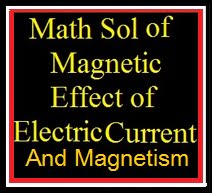# Math Solution of Magnetic Effect of Electric Current and Magnetism

Math Solution of Magnetic Effect of Electric Current and Magnetism. When electric current flows through a wire wound around an iron bar, the bar behaves like a magnet. This magnet is called an electromagnet. An electromagnet is formed due to the magnetic effect of electric current. This magnetic effect of electric current was discovered by Hans Christian Oersted. Once, while preparing for a lecture, he noticed that there was a deflection in the needle of a magnetic compass kept near a wire that was connected to a battery. This deflection occurred every time the battery was switched on and off. He realised that a magnetic field is created around a current-carrying wire in a circuit.

## Math Solution of Magnetic Effect of Electric Current and Magnetism

### Math Solution of Magnetic Effect of Electric Current and MagnetismMath Solution of Magnetic Effect of Electric Current and Magnetism